Bates College Calculus II Placement Exam

1. What is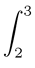3x2cos(x3)dx?   (1 point)

(a) sin 27 – sin 8

(b) 27 sin 27 – 8 sin 8

(c) cos 27 – cos 8

(d) 27 cos 27 – 8 cos 8

2. What is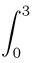xexdx?     (1 point)

(a) 4.5e3

(b) 2e3 + 1

(c) 4e3 – 1

(d) 3e3 – 3

3. What is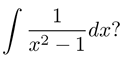(1 point)

(a) ln | x2 – 1 | + C

(b) ln | x – 1 | – ln | x + 1 | + C

(c)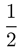(ln | x + 1 | – ln | x – 1 |) + C

(d)(ln | x – 1 | – ln | x + 1 |) + C

4. Which of the following is equal to the area between the curves y = x2– 1 and y = 4x – 4?      (1 point)

(a)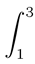[(x2 – 1) – (4x – 4)]dx

(b)[ (4x – 4) – ( x2 – 1) ]dx

(c)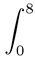[(x2 – 1) – (4x – 4)]dx

(d)[ (4x – 4) – ( x2 – 1) ]dx

5. Consider the region defined by y = e x, the x-axis, and the lines x = 1 and x = 3. Which of the following is equal to the volume obtained when this region is rotated about the x-axis?     (1 point)

(a)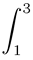ex dx

(b)(ex)2 dx

(c)πex dx

(d)π(ex)2 dx

6. Suppose that g(x) is increasing and concave down on the interval [a, b] and let I =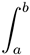g(x) dx. If L100, R100, and T100 are respectively the left-hand, right-hand, and trapezoidal sum approximations with 100 equal subdivisions for the integral I, which of the following must be true?      (1 point)

(a) L100 < I < T100 < R100

(b) L100< T100 < I < R100

(c) R100< I < T100 < L100

(d) R100 < T100 < I < L100

7. Consider the improper integral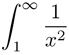. Which of the following is true?       (1 point)

(a) the integral converges to 0

(b) the integral converges to 0.5

(c) the integral converges to 1

(d) the integral diverges

8. What is the sum of the infinite series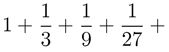. . . ?     (1 point)

(a) 0

(b) 1.5

(c) 2

(d) The series diverges

9) Consider the infinite series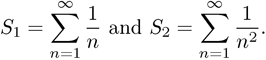Which of the following is true?   (1 Point)

(a) S1 converges and S2 converges

(b) S1 converges and S2 diverges

(c) S1 diverges and S2 converges

(d) S1 diverges and S2 diverges

10. Consider the infinite series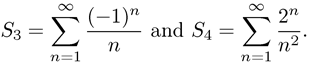Which of the following is true?   (1 Point)

(a) S3 converges and S4 converges

(b) S3 converges and S4 diverges

(c) S3 diverges and S4 converges

(d) S3 diverges and S4 diverges

11. Which of the following is the Taylor series about x = 0 for sin x?    (1 Point)

(a)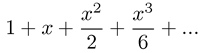(b)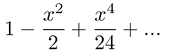(c)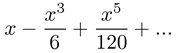(d)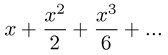12. Which of the following is the second degree Taylor polynomial about x = 4 for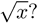(1 Point)

(a)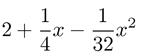(b)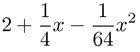(c)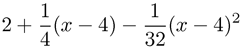(d)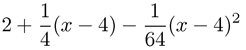13. What is the interval of convergence of the power series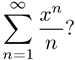(1 Point)

(a) – 1 < x < 1

(b) – 1 < x ≤ 1

(c) – 1 ≤ x < 1

(d) – 1 ≤ x ≤ 1

14. What is the solution to the differential equation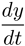= 6y that passes through the point (0, 2)?   (1 Point)

(a) y = 2e6t

(b) y = e6t + 1

(c) y = 3y2 + 2

(d) y = 3t2 + 2